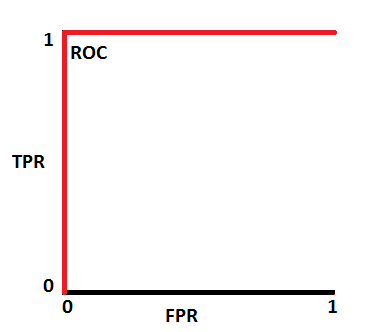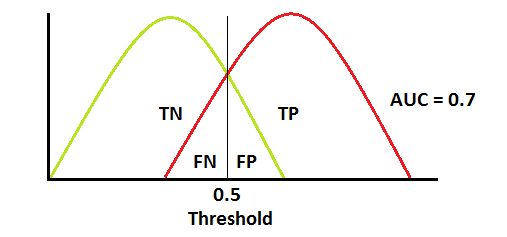top of page
Search

# AUC - ROC Curve in Machine Learning

In Machine Learning, performance measurement is an essential task. So when it comes to a classification problem, we can count on an AUC - ROC Curve. When we need to check or visualize the performance of the multi-class classification problem, we use the AUC (Area Under The Curve) ROC (Receiver Operating Characteristics) curve. It is one of the most important evaluation metrics for checking any classification model’s performance. It is also written as AUROC (Area Under the Receiver Operating Characteristics)

### What is the AUC - ROC Curve?

AUC - ROC curve is a performance measurement for the classification problems at various threshold settings. ROC is a probability curve and AUC represents the degree or measure of separability. It tells how much the model is capable of distinguishing between classes. Higher the AUC, the better the model is at predicting 0 classes as 0 and 1 classes as 1. By analogy, the Higher the AUC, the better the model is at distinguishing between patients with the disease and no disease.

The ROC curve is plotted with TPR against the FPR where TPR is on the y-axis and FPR is on the x-axis.AUC - ROC Curve [Image 2] (Image courtesy: My Photoshopped Collection)

### Defining terms used in AUC and ROC Curve.

TPR (True Positive Rate) / Recall /SensitivitySpecificityFPR### How to speculate about the performance of the model?

An excellent model has AUC near to the 1 which means it has a good measure of separability. A poor model has an AUC near 0 which means it has the worst measure of separability. In fact, it means it is reciprocating the result. It is predicting 0s as 1s and 1s as 0s. And when AUC is 0.5, it means the model has no class separation capacity whatsoever.

Let’s interpret the above statements.

As we know, ROC is a curve of probability. So let's plot the distributions of those probabilities: Note: Red distribution curve is of the positive class (patients with disease) and the green distribution curve is of the negative class(patients with no disease).This is an ideal situation. When two curves don’t overlap at all means model has an ideal measure of separability. It is perfectly able to distinguish between positive class and negative class.When two distributions overlap, we introduce type 1 and type 2 errors. Depending upon the threshold, we can minimize or maximize them. When AUC is 0.7, it means there is a 70% chance that the model will be able to distinguish between positive class and negative class.This is the worst situation. When AUC is approximately 0.5, the model has no discrimination capacity to distinguish between positive class and negative class.When AUC is approximately 0, the model is actually reciprocating the classes. It means the model is predicting a negative class as a positive class and vice versa.

### The relation between Sensitivity, Specificity, FPR, and Threshold.

Sensitivity and Specificity are inversely proportional to each other. So when we increase Sensitivity, Specificity decreases, and vice versa.

### Sensitivity⬆️, Specificity⬇️ and Sensitivity⬇️, Specificity⬆️

When we decrease the threshold, we get more positive values thus it increases the sensitivity and decreasing the specificity.

Similarly, when we increase the threshold, we get more negative values thus we get higher specificity and lower sensitivity.

As we know FPR is 1 - specificity. So when we increase TPR, FPR also increases and vice versa.

### How to use the AUC ROC curve for the multi-class model?

In a multi-class model, we can plot the N number of AUC ROC Curves for N number classes using the One vs ALL methodology. So for example, If you have three classes named X, Y, and Z, you will have one ROC for X classified against Y and Z, another ROC for Y classified against X and Z, and the third one of Z classified against Y and X.

Source: Towards Data Science

The Tech Platform